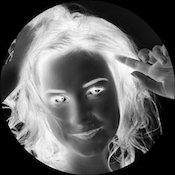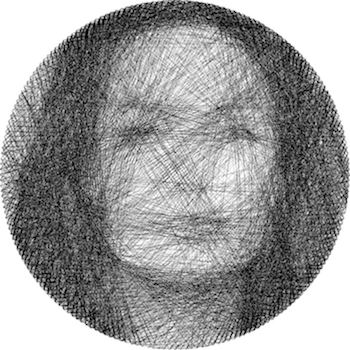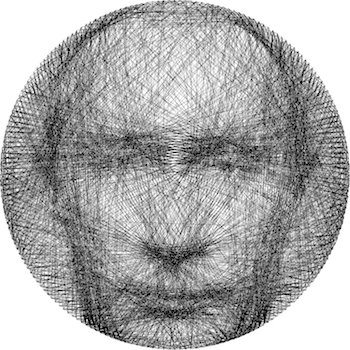# Thevelop

Not too long ago I came across a piece of art made by Petros Vrellis. A piece of art that makes the engineer in me scream: automation. As can be seen below Petros uses a circular loom and a lot of patience to develop portraits from thread. Below I’ll quickly go through the steps of automating the process but of course all the credits go to the artist behind this amazing idea! The final result is a halftone representation of an image using nothing but thread.The main idea is comprised of three steps. First a little image pre-processing is done to various input images for threading. Second is some processing done in python to determine where one should place the successive threads as to obtain the best image representation. Third, I can put my laserless lasercutter to work to do the heavy lifting.

## Image pre-processing

To allow image processing on a pixel level we use openCV. Let’s get started and do some initial processing of the image. The snippet below shows how one can load, crop and resize an image.

``````import cv2
import numpy as np

# Invert grayscale image
def invertImage(image):
return (255-image)

# Apply circular mask to image

return image

# Crop image
height, width = image.shape[0:2]
minEdge= min(height, width)
topEdge = int((height - minEdge)/2)
leftEdge = int((width - minEdge)/2)
imgCropped = image[topEdge:topEdge+minEdge, leftEdge:leftEdge+minEdge]

# Convert to grayscale
imgGray = cv2.cvtColor(imgCropped, cv2.COLOR_BGR2GRAY)

# Resize image

# Invert image
imgInverted = invertImage(imgSized)

``````

Once the image is of the correct size it is converted to a gray-scale image and inverted. In this form the image can be represented as an array where the originally darkest places have the highest value. Finally the image is masked with a circular mask (all values outside the circle are set to zero).Deciding where one needs to pass the thread as to obtain a representation of the original image is the main focus of the algorithm. For this the assumption is made that a circular loom is used with an evenly spaced amount of pins.

As seen in the clip above the algorithm starts at a random pin. It checks all possible options for the one that would best fit the image. It now draws the optimal line, accounts for the “darkness” added by this line and starts again. The functions below generate the pin coordinates of the loom and a mask representing a certain line.

``````# Compute coordinates of loom pins
def pinCoords(radius, nPins=200, offset=0, x0=None, y0=None):
alpha = np.linspace(0 + offset, 2*np.pi + offset, nPins + 1)

if (x0 == None) or (y0 == None):

coords = []
for angle in alpha[0:-1]:

coords.append((x, y))
return coords

def linePixels(pin0, pin1):
length = int(np.hypot(pin1 - pin0, pin1 - pin0))

x = np.linspace(pin0, pin1, length)
y = np.linspace(pin0, pin1, length)

return (x.astype(np.int)-1, y.astype(np.int)-1)
``````

Using the functions above the algorithm iteratively adds lines to the image until either the maximum number of lines is reached or the stopping criteria is reached. The fitness function is taken to be the specific line integral over the image. Lines that cover a lot of originally dark areas will therefore achieve a high fitness.

``````# Define pin coordinates

# Initialize variables
i = 0
lines = []
previousPins = []
oldPin = initPin

# Loop over lines until stopping criteria is reached
for line in range(nLines):
i += 1
bestLine = 0
oldCoord = coords[oldPin]

# Loop over possible lines
for index in range(1, nPins):
pin = (oldPin + index) % nPins

coord = coords[pin]
xLine, yLine = linePixels(oldCoord, coord)

# Fitness function

if (lineSum > bestLine) and not(pin in previousPins):
bestLine = lineSum
bestPin = pin

# Update previous pins
if len(previousPins) >= minLoop:
previousPins.pop(0)
previousPins.append(bestPin)

# Subtract new line from image

# Save line to results
lines.append((oldPin, bestPin))

# Break if no lines possible
if bestPin == oldPin:
break

# Prepare for next loop
oldPin = bestPin
``````

Once the algorithm no longer finds any possible lines the loop is broken and the result can be further processed. Below one can find two examples of the algorithms result. Move over the image in question to see the original image.